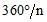# Periodicity: definition (structure)

## Definition

The Periodicity entity is defined by:

• a name
• a type of periodicity
• position of periodicity planes

## Name

The name to identify the periodicity is allocated automatically by Flux during the creation of this one.

The user cannot modify this name.

## Types of periodicity: 2D domain

The periodicity types and the characteristics useful for their description are gathered in the table below.

Translation Included length Offset position
along X along the X axis along the X axis
along Y along the Y axis along the Y axis
Rotation with angle Included angle of the domain Offset angle
about Z axis about Z axis with respect to the ZOX plane
Rotation with number of repetitions Number of repetitions Offset angle
about Z-axis along Z with respect to the ZOX plane

## Types of periodicity: 3D domain

The periodicity types and the characteristics useful for their description are gathered in the table below.

Translation Included length Offset position
along X along the X axis along the X axis
along Y along the Y axis along the Y axis
along Z along the Z axis along the Z axis
Rotation with angle Included angle of the domain Offset angle
Rotation with number of repetitions Number of repetitions Offset angle
about X-axis along X with respect to the XOY plane
about Y-axis along Y with respect to the YOZ plane
about Z-axis along Z with respect to the ZOX plane

## Composed periodicity (in 3D only)

The composed periodicity is a particular periodicity defined by the composition:

• of a rotation about an axis X (Y, Z respectively)
• of a symmetry with respect to the plane YOZ (ZOX, XOY respectively)

It is defined in the following manner:

• the first plane (P1) is defined by its angular position with respect to the main plane XOZ (XOY, ZOY respectively): offset angle (θ1)
• the second plane (P2) is defined by a rotation of plane P1 about Z-axis

(X-axis, Y-axis respectively): rotation angle (θ2 - θ1)

The rotation angle about the axis (θ2 - θ1) iswhere n is the number of repetitive patterns (repetitions).

• the third plane (P3) is defined by a symmetry of the plane P2 with respect to the symmetry plane: offset position of the symmetry plane on the Z rotation axis (X-axis, Y-axis respectively)## Important

It is not possible to change the type of the periodicity by editing its dialog box.

To change the periodicity type:

• Delete the periodicity
• Create a new periodicity

## Length and angle units

Length and angle units are those associated with the domain.

These units can be edited and modified in the dialog box of the Domain Type.